# What is written on Einstein's note?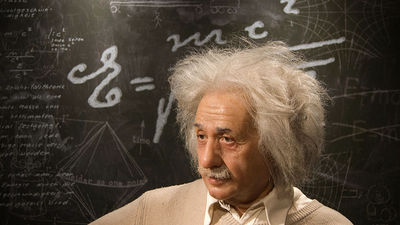BySebastian Niedlich

Speaking of Albert Einstein,General relativityIt is a theoretical physicist advocating various theories, including a synonym for the word "genius". The contents of such a note "Zurich · Note" written with precious private reasoning and calculation, which gives us a glimpse of a part of Einstein's thinking, is released.

Einstein's Zurich Notebook
http://www.pitt.edu/~jdnorton/Goodies/Zurich_Notebook/index.html

◆ Cover of notebook
The note has two covers. Einstein has written explanations for both of these, and the following cover was written by Einstein's secretary Helen Ducasse with a typewriter "Notes for Lectures on Relativity ... (note to explain the theory of relativity) The letter ''.And turning over this note will find "Relativity" and another cover written by Einstein himself.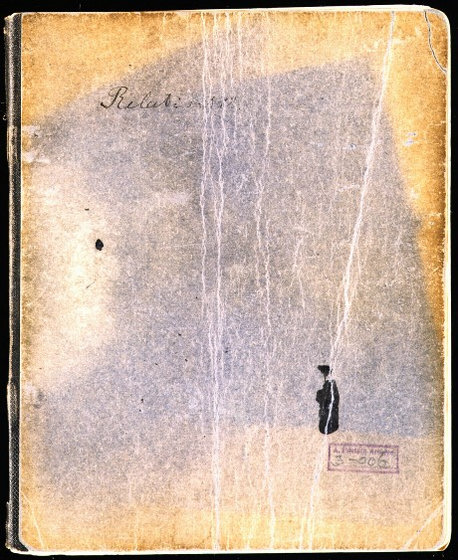And inside the cover written as "Relativity", there is a rough sketch like the following. These are all math puzzles.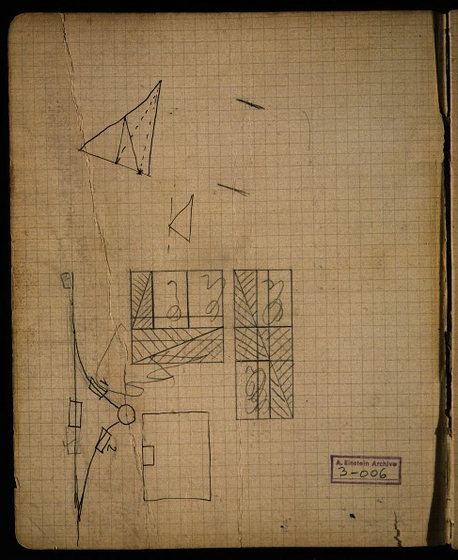The following figures are related to railway cars and branch roads running on rails, with rails as rails and rectangles as railway vehicles.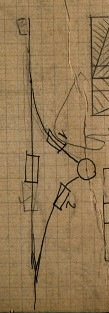The following figure where multiple squares and rectangles are arranged is a mathematics puzzle in which a square with a side length of "8" is replaced with a rectangle with a long side "13" short side "5". For a square with a side length of "8", the area is "8 × 8 = 64".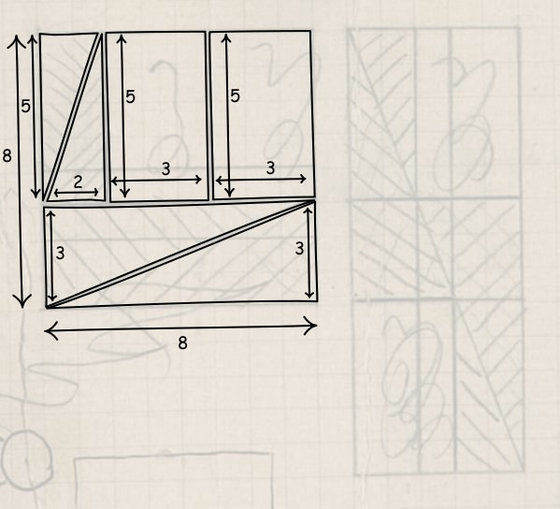However, the area of ​​the rectangle completed by finely dividing and rearranging the square is "13 × 5 = 65", and the area is increasing for some reason. Of course Einstein should have a solid understanding of this puzzle "Why do the area increase?", But truly mysterious is why Einstein was playing with such math puzzles. John D. Norton of the University of Pittsburgh, who explains each note, says "Maybe he was playing with someone's child."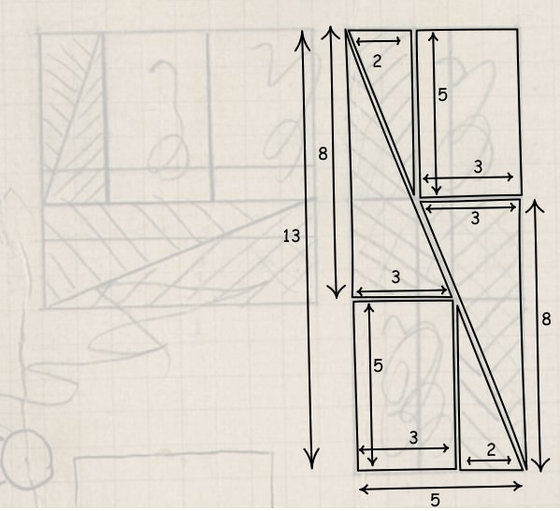The mysterious answer, in which the area becomes large if the square is rearranged into a rectangle,from hereYou can check.

◆ Four-dimensional electrodynamics
On this page you can see the theory of relativityMinkowskiThe four-dimensional approach of the element to electrodynamics in detail is described in detail.

Let the four-dimensional space be (x, y, z, ict) = (x1, x2, x3, x4)Four element vectorAnd 6 element vector, and how to calculate it.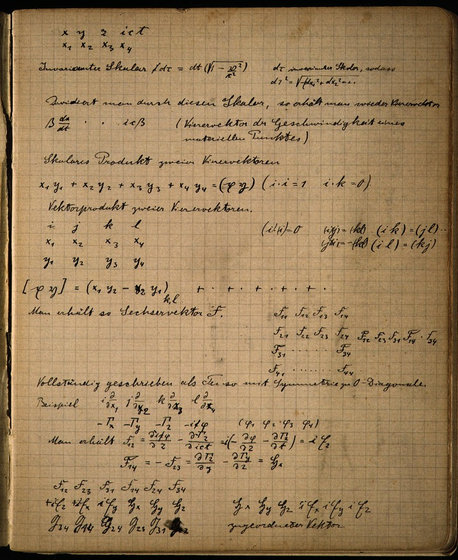◆ The first line of general theory of relativity
A page detailing the concepts of electrodynamics and thermophysics lasted about 13 pages ......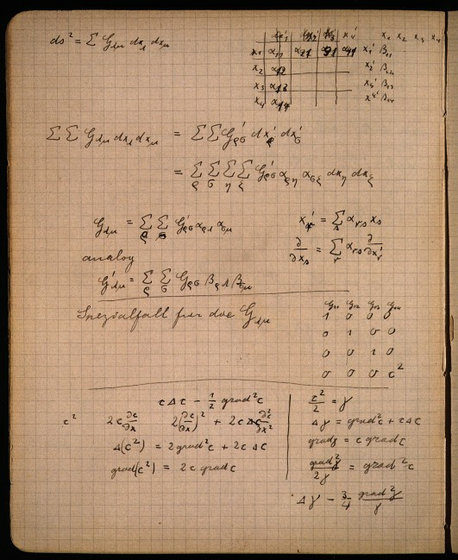And suddenly without any premonition, "Linear" which is the basic concept of general relativity is written on the top of the paper. About this, Mr. Norton says "This may be the case when Einstein wrote the first lineage."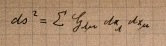The symbol "Gμν" in the above formula indicates that,Metrological tensor. This coefficient that appears several times in a note is changing to express it with lowercase "g" in Einstein's hands written several times. In addition, weighing tensor is said to be "gravity field method" based on "inverse square law of gravity".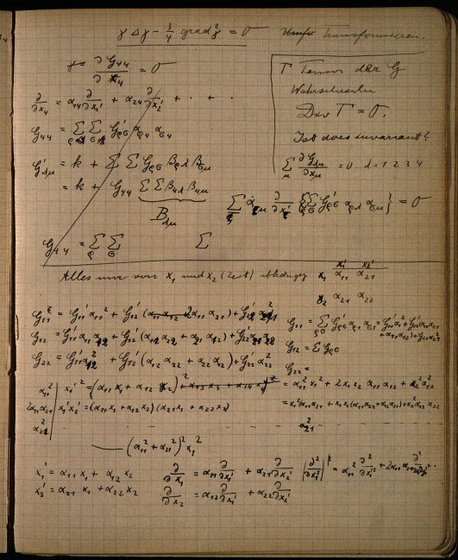In these pages where Einstein would have thought about "linear"Tensor analysis(PDF file)We do not use analysis methods such as.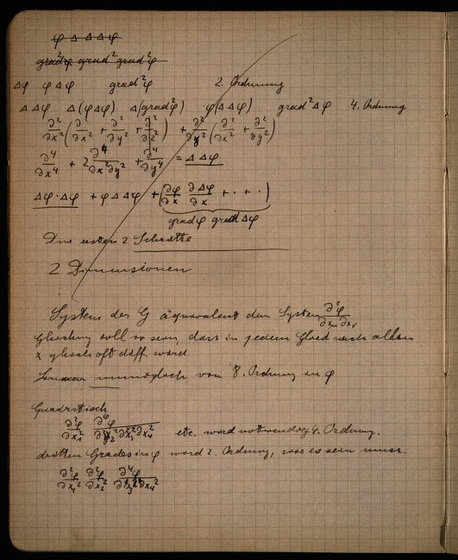◆ Curved surface motion
Analysis on curved movement is continued over 3 pages.

On these pages, Einstein has discovered some important concepts from classical physics. If an object moves on a curved surface according to inertia, the trajectory of an object moving on a curved surface indicates the shortest distance connecting two points on a curved surfaceGeodesic line.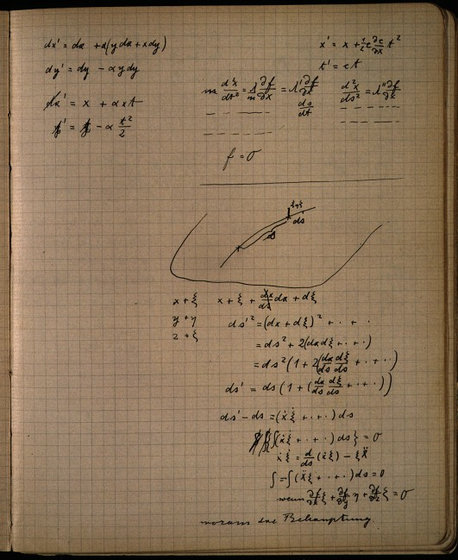A curved surface is defined by a scalar field "f", and an object moving on a curved surface can be expressed as an acceleration vector (d2x / dt2, d2y / dt2, d2z / dt2) by the equation of motion. Furthermore, since it is proportional to the reaction force of the curved surface, it can be expressed as (∂f / ∂x, ∂f / ∂y, ∂f / ∂z) from the slope of "f" It can be simplified.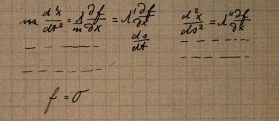◆ Other
Other statistics on thermal radiation of thermophysics ......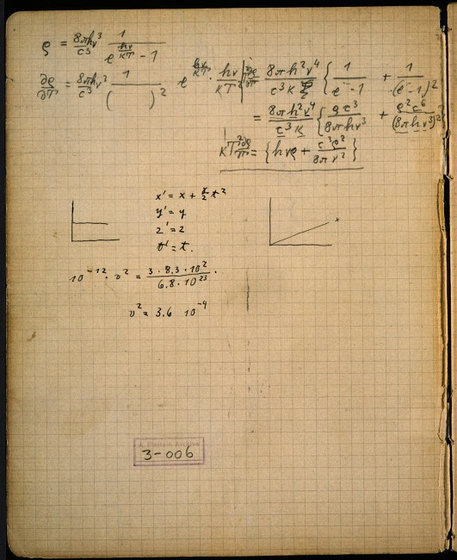Notes on universal gravitation of general relativity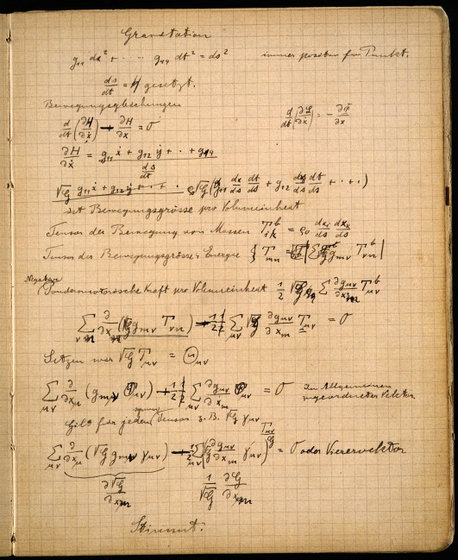Various calculation formulas are written such as consideration on metrological tensor.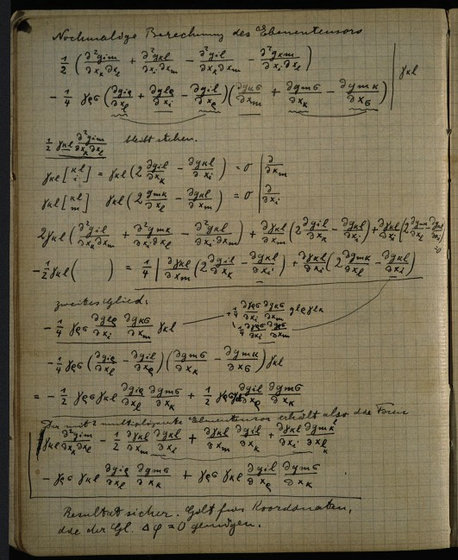As for the contents of mathematical expressions, even if it is almost chicken kanpan, I can imagine how Einstein was concentrating his heart on how to derive the theory of physics, provided that the content of private notebook is so much crowded with mathematical expressions.

in Note, Posted by logu_ii